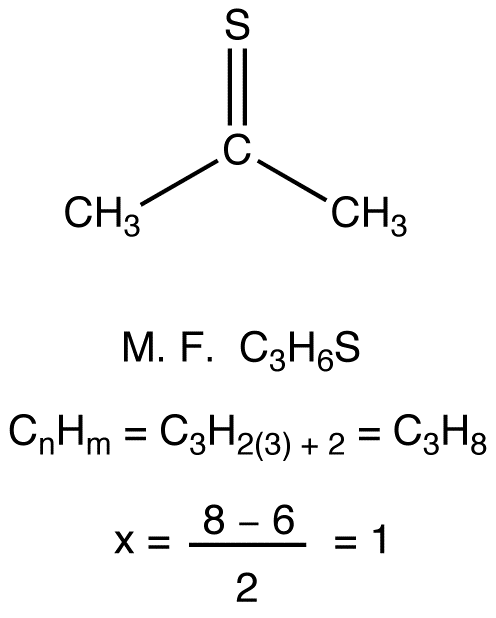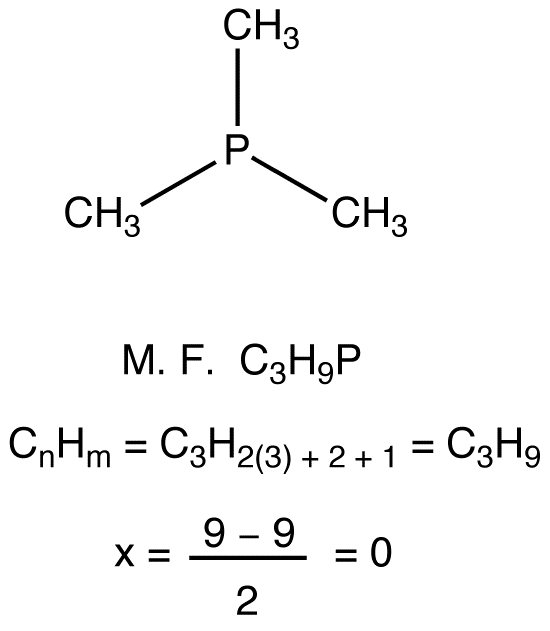# Degree of Unsaturation

•• Contributed by Gamini Gunawardena
• Associate Professor (Chemistry) at Utah Valley University

A number known as degree of unsaturation or index of hydrogen deficiency can be defined for any organic molecule. (In this writing, the symbol x is used to denote degree of unsaturation.)(If the molecule is resonance stabilized, this formula applies to the major resonance form.)If the structural formula of a compound is not known, but the molecular formula is, the degree of unsaturation of the compound can be calculated using the following general formula.(This method is limited to organic compounds containing carbon, hydrogen, oxygen, sulfur, nitrogen, phosphorus, and halogens, which are the most common elements in naturally occurring organic compounds.)

eg. 1:eg. 2:eg. 3:eg. 4:eg. 5:eg. 6:eg. 7:eg. 8: